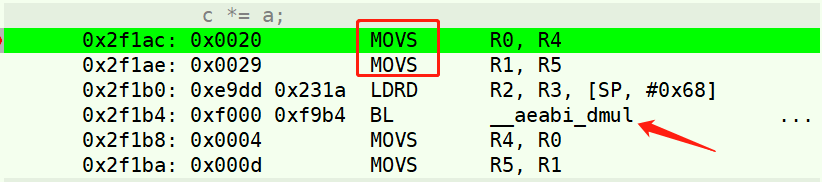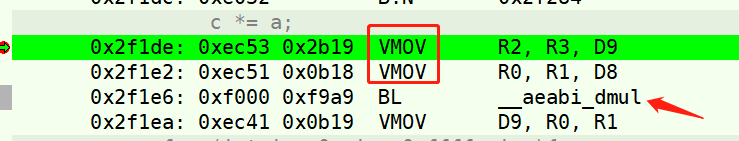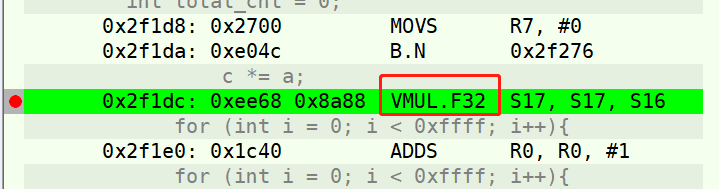# Cortex-M FPU的一点点小问题

FPU全称Float Point Unit，即浮点运算单元，是CPU中针对浮点运算进行加速的单元，在Cortex-M系列CPU中，FPU是一个可选选项，引入FPU之后可以有效加速浮点运算速度，提高CPU性能。

``````double a = 3.1415926;
double c = 1.0;
long long t = 0;
t = get_timer_value();
for(int i = 0; i < 0xffff; i++){
c *= a;
}
t = get_timer_value() - t;
printf("time = %d\r\n", t);````````````float a = 3.1415926;
float c = 1.0;
long long t = 0;
t = get_timer_value();
for(int i = 0; i < 0xffff; i++){
c *= a;
}
t = get_timer_value() - t;
printf("time = %d\r\n", t);``````1. 因为`<math.h>`库中类似`sin(), cos()`函数的实现也是Double类型的，所以这些函数依然是CPU执行的，和FPU没有关系，如果想用FPU，可以调用float类型版本的，也就是在后面加一个f后缀，比如`sinf(), cosf()`这样函数就可以调用FPU进行计算了！
2. 对于多任务或者中断中使用FPU的时候，要注意FPU是不支持重入的，简单来说就是FPU运算不能被打断后执行新的FPU运算，用户必须避免这种情形，而这条限制明显会增加代码复杂度。